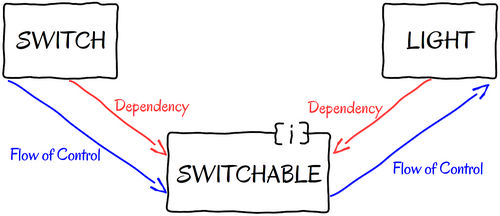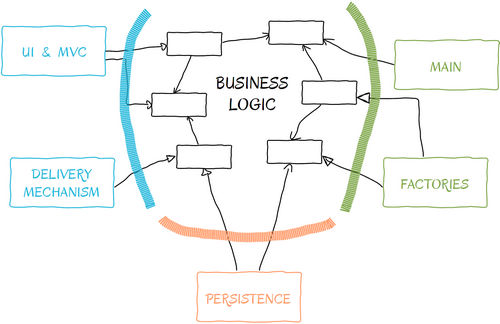求知 文章 文库 Lib 视频 iPerson 课程 认证 咨询 工具 讲座 Modeler Code分享到

1.内聚力

 ```class ANOTCohesiveClass { private \$firstNumber; private \$secondNumber; private \$length; private \$width; function __construct(\$firstNumber, \$secondNumber) { \$this->firstNumber = \$firstNumber; \$this->secondNumber = \$secondNumber; } function setLength(\$length) { \$this->length = \$length; } function setHeight(\$height) { \$this->width = \$height; } function add() { return \$this->firstNumber + \$this->secondNumber; } function subtract() { return \$this->firstNumber - \$this->secondNumber; } function area() { return \$this->length * \$this->width; } } ```

 ```class ACohesiveClass { private \$firstNumber; private \$secondNumber; function __construct(\$firstNumber, \$secondNumber) { \$this->firstNumber = \$firstNumber; \$this->secondNumber = \$secondNumber; } function add() { return \$this->firstNumber + \$this->secondNumber; } function subtract() { return \$this->firstNumber - \$this->secondNumber; } } ```

2.正交性

 ```class Calculator { private \$firstNumber; private \$secondNumber; function __construct(\$firstNumber, \$secondNumber) { \$this->firstNumber = \$firstNumber; \$this->secondNumber = \$secondNumber; } function add() { \$sum = \$this->firstNumber + \$this->secondNumber; if (\$sum > 100) { (new AlertMechanism())->tooBigNumber(\$sum); } return \$sum; } function subtract() { return \$this->firstNumber - \$this->secondNumber; } } class AlertMechanism { function tooBigNumber(\$number) { echo \$number . 'is too big!'; } } ```

 ```class Calculator { private \$firstNumber; private \$secondNumber; function __construct(\$firstNumber, \$secondNumber) { \$this->firstNumber = \$firstNumber; \$this->secondNumber = \$secondNumber; } function add() { return \$this->firstNumber + \$this->secondNumber; } function subtract() { return \$this->firstNumber - \$this->secondNumber; } } class AlertMechanism { function checkLimits(\$firstNumber, \$secondNumber) { \$sum = (new Calculator(\$firstNumber, \$secondNumber))->add(); if (\$sum > 100) { \$this->tooBigNumber(\$sum); } } function tooBigNumber(\$number) { echo \$number . 'is too big!'; } } ```

3.依赖和耦合

Coupling in a Field

 ```class Display { private \$calculator; function __construct() { \$this->calculator = new Calculator(1,2); } } ```

 ```class Display { private \$calculator; function __construct() { \$this->calculator = new Calculator(1, 2); } function printSum() { echo \$this->calculator->add(); } } ```

 ```class Display { private \$calculator; function __construct() { \$this->calculator = \$this->makeCalculator(); } function printSum() { echo \$this->calculator->add(); } function makeCalculator() { return new Calculator(1, 2); } } ```

 ```class AdvancedCalculator extends Calculator { function sinus(\$value) { return sin(\$value); } } ```

 ```class Display { private \$calculator; function __construct(Calculator \$calculator = null) { \$this->calculator = \$calculator ? : \$this->makeCalculator(); } // ... //. } ```

 ``` interface CanCompute { function add(); function subtract(); } class Calculator implements CanCompute { private \$firstNumber; private \$secondNumber; function __construct(\$firstNumber, \$secondNumber) { \$this->firstNumber = \$firstNumber; \$this->secondNumber = \$secondNumber; } function add() { return \$this->firstNumber + \$this->secondNumber; } function subtract() { return \$this->firstNumber - \$this->secondNumber; } } class Display { private \$calculator; function __construct(CanCompute \$calculator = null) { \$this->calculator = \$calculator ? : \$this->makeCalculator(); } function printSum() { echo \$this->calculator->add(); } function makeCalculator() { return new Calculator(1, 2); } } ```

Display构造函数期望有个对象来实现Cancompute接口，这时，Display的依赖对象Calculator被打破。然而，我们可以创建另一个类对象来实现Cancompute，并且传递一个对象到Display的构造函数中。Display现在只依赖于Cancompute接口，但即使这样依赖关系仍然是可选的。如果我们不传递任何参数给Display的构造函数，那么它将通过调用makeCalculator()方法来创建一个Calculator对象。这种技术经常被开发者们使用，尤其对驱动测试开发（TDD）极其有帮助。

SOLID原则

SOLID是一套代码编写守则，也就是大家常常说的敏捷开发原则，最初由Robert C. Martin所提出。使用它编写出来的代码不仅干净整洁，而且易维护、易修改和易扩展。实践表明，其在可维护性上有着非常积极的影响，更多资料大家可以阅读： Agile Software Development, Principles, Patterns, and Practices

SOLID所涵盖的话题非常广，下面我将会针对本文的主旨介绍一些简单易学的方法。

1.单一责任原则（SRP）

 ```class Reporter { function generateIncomeReports(); function generatePaymentsReports(); function computeBalance(); function printReport(); } ```

2.Open-Closed原则（OCP）

 ```class Switch_ { private \$fan; function __construct() { \$this->fan = new Fan(); } function turnOn() { \$this->fan->on(); } function turnOff() { \$this->fan->off(); } } ```

 ```interface Switchable { function on(); function off(); } class Fan implements Switchable { public function on() { // code to start the fan. } public function off() { // code to stop the fan. } } class Switch_ { private \$switchable; function __construct(Switchable \$switchable) { \$this->switchable = \$switchable; } function turnOn() { \$this->switchable->on(); } function turnOff() { \$this->switchable->off(); } } ```

 ```class Light implements Switchable { public function on() { // code to turn ligh on. } public function off() { // code to turn light off. } } class SomeWhereInYourCode { function controlLight() { \$light = new Light(); \$switch = new Switch_(\$light); \$switch->turnOn(); \$switch->turnOff(); } } ```

3.Liskov替换原则（LSP）

LSP是指子类永不打破父类的功能，这点是非常重要的。用户定义一个子类只是希望能实现其自有功能，而不是去影响原来的功能。

 ```class Rectangle { private \$width; private \$height; function setWidth(\$width) { \$this->width = \$width; } function setHeigth(\$heigth) { \$this->height = \$heigth; } function area() { return \$this->width * \$this->height; } } ```

 ```class Geometry { function rectArea(Rectangle \$rectangle) { \$rectangle->setWidth(10); \$rectangle->setHeigth(5); return \$rectangle->area(); } } ```

rectArea()方法接受一个Rectangle对象作为一个参数，设置其高度和宽度并且返回该图形的面积。

 ```class Square extends Rectangle { // What code to write here?. } ```

 ```class Rectangle { protected \$width; protected \$height; // ... //. } class Square extends Rectangle { function area() { return \$this->width ^ 2; } } ```

4.接口隔离原则（ISP

 ```interface Broker { function buy(\$symbol, \$volume); function sell(\$symbol, \$volume); function dailyLoss(\$date); function dailyEarnings(\$date); } class NYSEBroker implements Broker { public function buy(\$symbol, \$volume) { // implementsation goes here. } public function currentBalance() { // implementsation goes here. } public function dailyEarnings(\$date) { // implementsation goes here. } public function dailyLoss(\$date) { // implementsation goes here. } public function sell(\$symbol, \$volume) { // implementsation goes here. } } class TransactionsUI { private \$broker; function __construct(Broker \$broker) { \$this->broker = \$broker; } function buyStocks() { // UI logic here to obtain information from a form into \$data. \$this->broker->buy(\$data['sybmol'], \$data['volume']); } function sellStocks() { // UI logic here to obtain information from a form into \$data. \$this->broker->sell(\$data['sybmol'], \$data['volume']); } } class DailyReporter { private \$broker; function __construct(Broker \$broker) { \$this->broker = \$broker; } function currentBalance() { echo 'Current balace for today ' . date(time()) . "\n"; echo 'Earnings: ' . \$this->broker->dailyEarnings(time()) . "\n"; echo 'Losses: ' . \$this->broker->dailyLoss(time()) . "\n"; } } ```

 ```interface BrokerTransactions { function buy(\$symbol, \$volume); function sell(\$symbol, \$volume); } interface BrokerStatistics { function dailyLoss(\$date); function dailyEarnings(\$date); } class NYSEBroker implements BrokerTransactions, BrokerStatistics { public function buy(\$symbol, \$volume) { // implementsation goes here. } public function currentBalance() { // implementsation goes here. } public function dailyEarnings(\$date) { // implementsation goes here. } public function dailyLoss(\$date) { // implementsation goes here. } public function sell(\$symbol, \$volume) { // implementsation goes here. } } class TransactionsUI { private \$broker; function __construct(BrokerTransactions \$broker) { \$this->broker = \$broker; } function buyStocks() { // UI logic here to obtain information from a form into \$data. \$this->broker->buy(\$data['sybmol'], \$data['volume']); } function sellStocks() { // UI logic here to obtain information from a form into \$data. \$this->broker->sell(\$data['sybmol'], \$data['volume']); } } class DailyReporter { private \$broker; function __construct(BrokerStatistics \$broker) { \$this->broker = \$broker; } function currentBalance() { echo 'Current balace for today ' . date(time()) . "\n"; echo 'Earnings: ' . \$this->broker->dailyEarnings(time()) . "\n"; echo 'Losses: ' . \$this->broker->dailyLoss(time()) . "\n"; } } ```

5.依赖倒置原则（DIP

DIP的诀窍是你想反转依赖，但是又想一直保持着整个控制流。回顾下OCP（Switch和Light类），在原始实现中是直接利用开关来控制灯的。相关文章 深度解析：清理烂代码 如何编写出拥抱变化的代码 重构-使代码更简洁优美 团队项目开发"编码规范"系列文章
 相关文档 重构-改善既有代码的设计 软件重构v2 代码整洁之道 高质量编程规范
 相关课程 基于HTML5客户端、Web端的应用开发 HTML 5+CSS 开发 嵌入式C高质量编程 C++高级编程

 分享到

 WEB应用程序UI模版代码编写 C# 编码规范和编程好习惯 什么是防御性编程 善于防守-健壮代码的防御性 Visual C++编程命名规则 JavaScript程序编码规范

 设计模式原理与应用 从需求过渡到设计 软件设计原理与实践 如何编写高质量代码 单元测试、重构及持续集成 软件开发过程指南

 某全球知名通信公司 代码整洁 横河电机 如何编写高质量代码 某知名金融软件服务商 代码评审 东软集团 代码重构 某金融软件服务商 技术文档 中达电通 设计模式原理与实践 法国电信 技术文档编写与管理